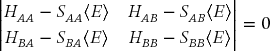# Molecular bonding and structure

Molecular bonding and structure

Bonding and the changes in bonding that occur in molecular encounters are obviously at the heart of chemistry. We have covered a lot of ground in chemistry without every really defining chemical bonds more precisely than involving either the sharing of electrons (covalent bonding) or the binding of ions by electrostatic interactions (ionic bonding). In Chapter 4, we investigated all kinds of weaker intermolecular interactions, for example, hydrogen bonding and multipole interactions. We have discussed electrostatic interactions, which hold together ionic solids and are essential for understanding electrolyte solutions, in Chapter 14. While we have a good idea that covalent bonding – the most prevalent form of bonding in molecules and which is also important in many solids – involves the sharing of electrons, we have yet to really understand it or treat it fundamentally. This is because we need to understand quantum mechanics first before we can really approach covalent bonding.

There are several flavors of covalent bonds. We will start out discussing two-center bonding. A single bond between two H atoms in H2, of course, is the simplest case and the place to start. Two-center bonding is also extremely common – at least, many bonds are predominantly two-center. However, there are many counter examples. You have encountered delocalized bonding in conjugated organic molecules such as benzene. You may have encountered three-center bonding in boron hydrides and some compounds involving halogens, such as HF2. Metallic bonding is also highly delocalized. In two-center bonding we generally think of two atoms coming together, each contributing an electron, then sharing these electrons to form a bond. If the electrons are shared equally, the bond is nonpolar. If the sharing is unequal, the bond is polar. A dative bond is a type of covalent bond formed when both electrons are contributed by one atom. This concept is common in coordination chemistry. Once we have a clearer picture of what molecular orbitals are and discover how molecules minimize their energies by filling them and adjusting their structure, we will see that these semantic distinctions are sometimes just that. However, as we have learned from our introduction to quantum mechanics, part of our learning how to understand the interactions of subatomic particles, atoms and molecules is learning how to graft classical terms and analogies onto intrinsically quantum phenomena.

## 24.1 Born–Oppenheimer approximation

To write the Hamiltonian of a molecule we add the potential energy to the kinetic energy. Contributions to the sum are made by both the electrons and the nuclei. The kinetic energy is a simple sum of an electronic term Te and a nuclear term Tn. The potential energy has contributions from the repulsive electron–electron interactions Vee and internuclear interactions Vnn, as well as the attractive electron–nuclei term Ven. Thus, the total Hamiltonian is

(24.1)Nuclei are much heavier and faster than electrons. Therefore, we can treat the nuclei as fixed and separate nuclear motion from electronic motion. This means that the electrons react so quickly to changes in nuclear positions, that they are always able to relax to their lowest energy configuration for any arrangement of the nuclei. This approximation was proposed by Max Born and J. Robert Oppenheimer.1 It is a good approximation for ground-state molecules and most excited states. Some excited states of polyatomic molecules and the electronic states of cations are where violations are most likely to be found. The Born–Oppenheimer approximation allows us to construct molecular potential energy curves and potential energy surfaces (PESs) using the following procedure.

For fixed nuclei, the nuclear kinetic energy term is zero and the internuclear potential is constant. There exists a set of electronic wavefunctions that satisfy the electronic Schrödinger equation

(24.2)where

(24.3)The electronic wavefunctions ψe depend on the nuclear coordinates. However, the Born–Oppenheimer approximation allows us to use these coordinates as a parameter. The nuclear coordinates are set to a given value and the Schrödinger equation is solved in the electronic coordinates to arrive at a value of the total energy. The procedure is repeated until we have calculated values for the energy for every value of the nuclear coordinates. For a diatomic molecule such as SrH – a random example depicted in Fig. 24.1 – this corresponds to calculating the energy as a function of the internuclear distance between the Sr atom and the H atom. The results of such a calculation are shown in Fig. 24.1. The ground state, denoted with X, is bound and has a well depth of De. A bound electronically excited state, denoted C in this example, is also shown.Figure 24.1 Born–Oppenheimer potential energy curves for the ground state of SrH, also called the X state, and one particular excited state designated as the C state. Total energy is plotted along the y-axis and the internuclear distance between the Sr and H nuclei is plotted along the x-axis. The horizontal lines represent different vibrational levels within each electronic state. The lowest energy vibrational state is labeled with the vibrational quantum number v = 0. D0 is the dissociation energy from the v = 0 level. De is the dissociation energy measured from the minimum of the potential energy curve.

As far as the motion of the nuclei is concerned, the rapid response of the electrons means that the electronic energy Ee can be treated as part of the potential field in which the nuclei move. The nuclear Hamiltonian is then

(24.4)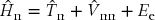and the Schrödinger equation for nuclear motion,

(24.5)can be solved independently for the nuclear wavefunctions ψn and the nuclear energy En. The total wavefunction can be factored and is given by the product of the electronic and nuclear wavefunctions,

(24.6)where τe and τn are the electronic and nuclear coordinates, respectively. The energy of such a factored wavefunction is given by the sum of electronic and nuclear contribution,

(24.7)To a first approximation, the vibrational and rotation degrees of freedom are independent. Therefore, the nuclear wavefunction can be further factored into vibrational and rotational components, and the energy is given by the sum of vibrational and rotational energies. In summary,

(24.8)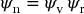(24.9)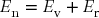(24.10)(24.11)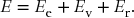We will find that to, a first approximation, the vibrational contribution can be treated as a harmonic oscillator near the ground state and then as a Morse oscillator closer to the dissociation limit. The rotational contribution is that of a rigid rotor with centrifugal distortion added at high levels of excitation. We will return to these components in detail when we discuss molecular spectroscopy in the next chapter. For now, we concentrate on the electronic term and how it is related to bonding.

## 24.2 Valence bond theory

How is a chemical bond formed? The first successful quantum mechanical theory of bond formation was the valence bond theory, in which electrons occupying atomic orbitals in the constituent atoms are brought together and combined either by adding or subtracting their wavefunctions. In constructing the wavefunctions that describe the combination of two orbitals we observe several things:

• A bond is formed by combining atomic orbitals from the two atoms that are bonding.
• Hybridization is introduced to explain the bonding of polyatomic molecules.
• The orbitals form a bonding combination by enhancing electron density between the nuclei caused by favorable orbital overlap (constructive interference between constituent orbitals).
• The orbitals form an antibonding combination when electron density is insufficiently built up between the nuclei (destructive interference between constituent orbitals).
• The complete wavefunction is composed of a spatial part multiplied by a spin part.
• Electrons must be paired so that they obey the Pauli exclusion principle – no two electrons have the same quantum numbers. The total wavefunction must be antisymmetric with respect to particle exchange.
• Good wavefunctions form an orthonormal set.
• σ bonds are axially symmetric and exhibit no nodes.
• π bonds are antisymmetric about a nodal plane containing the bond axis.
• Antibonding orbitals (either σ or π) have a nodal plane perpendicular to the internuclear axis along the bond.

### 24.2.1 Homonuclear diatomic molecules

We start by trying to form an H2 molecule from two H atoms – HA and HB – each with one electron in a 1s orbital. We will attempt to construct a wavefunction that describes the system in terms of the two wavefunctions that describe the atoms. The system is the sum of two electrons and two protons placed in the same region of space. Nuclei of like atoms are indistinguishable, just as are all electrons. When we bring two atoms together, we cannot distinguish whether it is electron 1 or electron 2 that occupies the orbital on atom A, and vice versa for atom B. We account for this in the system wavefunction by allowing for both possibilities in the spatial part of the wavefunction

(24.12)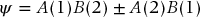where A is a 1s orbital on atom HA and B is a 1s orbital on atom HB. The (+) combination corresponds to constructive interference between the atomic wavefunctions. We will see that this forms the bonding combination. The (–) combination corresponds to the antibonding combination formed by destructive interference of the wavefunctions in the internuclear region.

To complete the wavefunction we now multiply the spatial part by the spin component. We have already encountered the four combinations of two spin states when we dealt with making proper wavefunctions for multi-electron atoms. The same combination rules are applicable to molecules,

(24.13)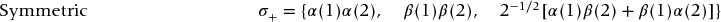(24.14)To couple the proper spin component with the proper spatial component, we consider the symmetry upon electron exchange

(24.15)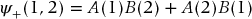(24.16)(24.17)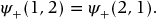Therefore, ψ+(1,2) is symmetric with respect to electron exchange. For the minus combination,

(24.18)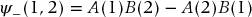(24.19)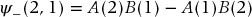(24.20)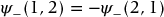Therefore ψ(1,2) is antisymmetric with respect to electron exchange.

The total wavefunction is formed from the product of the spatial and spin parts and must be antisymmetric. Therefore, the proper full wavefunctions are

(24.21)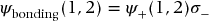(24.22)There is only one way to write the bonding wavefunction. It corresponds to a singlet state. There are three ways to write the antibonding wavefunction. These correspond to three degenerate states for this one-electron configuration, a triplet state. Degenerate states have the same energy.

Bonds and orbitals are classified based on the symmetry of the spatial distribution of electrons in the bond or orbital. A σ bond has cylindrical symmetry about the internuclear axis and no nodal plane along this axis, as shown in Fig. 24.2(a). A π bond has a nodal plane containing the internuclear axis, as in Fig. 24.2(b).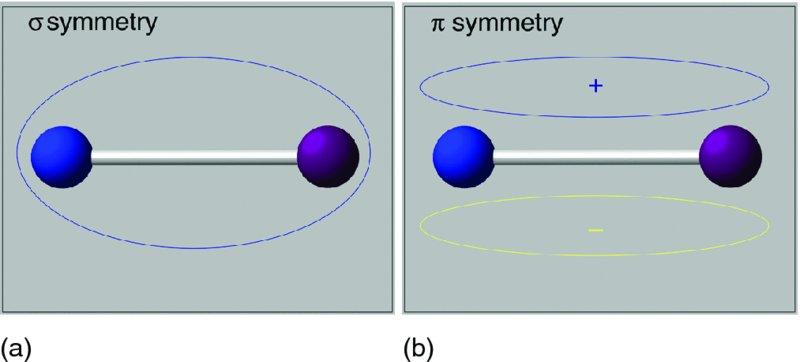Figure 24.2 (a) An example of a bonding orbital that has σ symmetry. (b) An example of a bonding orbital that has π symmetry.

The presence of nodal planes is not the only way to characterize orbitals. Three symmetry operations are considered when naming orbitals:

1. Reflection in a plane placed at the midpoint between nuclei and perpendicular to the internuclear axis.
2. Reflection in a plane in which the internuclear axis lies.
3. Inversion through the midpoint on the internuclear axis.

A diatomic molecule also contains an axis of rotational symmetry along the internuclear axis (it is a C axis, more on that in the next chapter). This is not considered in the symmetry designation of orbitals. However, it does define the vertical plane. The vertical plane is defined as the plane containing the highest-fold rotational axis, which in this case is the internuclear axis. Reflection is denoted by the operator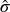(sorry, some letters are too popular). Thus, reflection in the vertical plane (the one containing the internuclear axis) is. This is the operation defined in item (2). Reflection in a plane perpendicular to the internuclear axis is denoted reflection through a horizontal plane with operator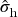. Inversion is denoted by the operator. All three symmetry operations are denoted in Figure 24.3.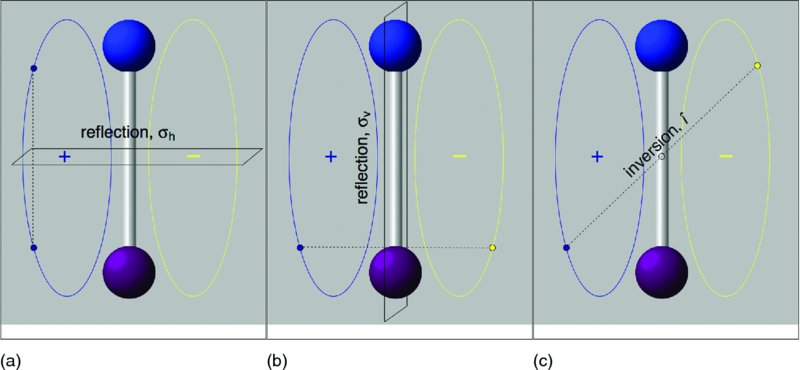Figure 24.3 Symmetry elements commonly encountered in diatomic molecules. (a) Reflection through a horizontal plane denoted by the operator . (b) Reflection through a vertical plane denoted by the operator . (c) Inversion through a center of inversion denoted by the operator .

When a symmetry operation acts on an object, the object must be transformed into a configuration that is indistinguishable from the original configuration apart from its sign. For example, the symbol ↔ is symmetric upon rotation by π radians about an axis perpendicular to the page (a C2 rotation). This rotation is a symmetry operation of the symbol. Rotation by only π/2 leads to ↕. This is distinguishable from the original and does not correspond to a symmetry operation of the object. If an object does not exhibit a certain symmetry element it is asymmetric with respect to that symmetry element. If the object is exactly the same after application of the symmetry operation, the object is symmetric with respect to the symmetry element. For example,is transformed to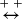by a C2 rotation, the plus signs indicating that the two ends of the arrow both have the same sign. An object that is exactly the same apart from a change in sign after the application of a symmetry operation is said to antisymmetric. For example,is transformed into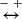by a C2 rotation. This object – with opposite signs at the two ends – is antisymmetric with respect to the symmetry operation.

Let us apply this to diatomic molecules. We are concerned with the symmetry of the wavefunctions formed by the bonding and antibonding combinations. The three cases described above are represented by the relations

(24.23)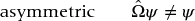(24.24)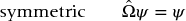(24.25)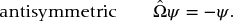The last two equations represent eigenvalue equations. The symmetry operators have eigenvalues of either 1 or –1. In Eq. (24.23) the wavefunction does not correspond to an eigenfunction of the symmetry operator. The symmetry with respect to inversion determines whether a wavefunction is gerade (German for even) or ungerade (odd). Gerade symmetry corresponds to(no change in sign upon inversion) and is denoted g. Ungerade symmetry corresponds(change in sign upon inversion) and is denoted u.

Antibonding orbitals contain a nodal plane perpendicular to the internuclear axis. This corresponds to aoperation. Since the wavefunction changes sign on either side of a nodal plane, we see thatcorresponds to an antibonding orbital andcorresponds to a bonding orbital.

The final element in the designation of orbitals does not involve symmetry elements; rather, it is based upon the electron angular momentum. Just as we had s, p, d, f, … atomic orbitals, we designate the orbitals in molecules with (switching to the Greek alphabet) σ, π, δ, φ, …. In atoms, the letter designation was determined by orbital angular momentum quantum number l. In molecules, the letter designation is determined by the projection of the angular momentum along the internuclear axis, which also takes on integer values 0, 1, 2, 3, … in units of ℏ. In your garden-variety organic molecules, only σ and π orbitals are encountered. Involving higher-Z atoms can lead to the formation of δ or even φ orbitals, though higher projections of angular momentum become progressively less likely.

### 24.2.2 Polyatomic molecules

For polyatomic molecules in the valence bond description, we think of two or more orbitals from one of the atoms undergoing the process of hybridization or promotion to form a set of hybrid orbitals. This is how we form the familiar sp, sp2 and sp3 hybrids of the C atom. A variety of hybridization schemes have been rationalized. These are illustrated in Table 24.1.

Hybridization does not happen and valence bond theory is rather inaccurate. A carbon atom simply does not have a conversation with itself, think that it wants to bond with four H atoms, spontaneously form four sp3 orbitals, and then have these hybrid orbitals form bonds with the individual H atoms along the way to making CH4. Nonetheless, both the concept of hybridization and the valence bond approach are highly successful and useful schemes. How can that be? Remember what we said during the construction of Old Quantum Theory, we will use classical analogies and push them as far as we can. Hybridization accepts a quantum idea (atomic orbitals) and tries to push it into an explanation of molecules. We need to be able to collect our thoughts and conceptions of what a covalent bond is. With our vocabulary drawn from valence bond theory and hybridization we can make a first-order approximation toward a more well-defined concept of what a covalent bond is. This puts us in a position to explore how we can improve upon these theoretical underpinnings.

## 24.3 Molecular orbital theory

What does an atomic orbital have to do with a molecule or a molecular bond? An atomic orbital is related to the wavefunction that describes the result of an atomic potential. A molecule has a potential that is constructed from the sum of the interactions of all the nuclei and all the electrons. For the valence electrons, this potential does not look anything like a central-field-atomic potential. Instead of producing atomic orbital states, a molecular potential forms molecular orbitals. Rather than confining bonding to a conception of hybrid orbitals centered on atoms that combine only by addition or subtraction between two centers, imagine now that we allow the electrons to occupy orbitals that can spread throughout the molecule and occupy the space that allows them to lower their energy as fully as possible. The resulting molecular orbitals will often be localized between atoms approximating a two-center bond. They are then associated with one particular bond. However, we do not a priori constrain them to do so.

To make a bond with molecular orbital theory, we generalize the procedure of valence bond theory by recognizing that it is the molecular potential that determines the nature of molecular orbitals in the valence region.

• A bond is formed by combining two or more basis functions.
• The basis functions can correspond to atomic orbitals, but they do not have to.
• The resulting orbitals are not constrained to be between only two atoms but can extend over the whole molecule.
• We define the Hamiltonian and solve for the best linear combination wavefunctions that describe the system, that is, give the lowest energy.
• The wavefunctions will be antisymmetric with respect to electron exchange, orthonormal, and obey the Pauli exclusion principle.
• Bonding, antibonding and nonbonding combinations are possible.

The strategy is pretty clear. Define the Hamiltonian then attempt to find solutions in terms of well-defined basis functions. Early work concentrated on using atomic orbitals as the basis set. This led to the idea of finding solutions in terms of linear combinations of atomic orbitals (LCAO), or LCAO-MO theory. Such orbitals are also known as Slater-type orbitals (STO), and are intuitive and familiar but difficult computationally. The functions in atomic orbitals are difficult to integrate, and we need to integrate to calculate expectation values. Therefore, an alternative is to abandon atomic orbitals and instead use a functional form that is easy to integrate and still localized about the atoms. For this, Gaussian-type orbitals (GTO) or variations on Gaussian functions are chosen. These do not correspond to atomic orbitals but they are much easier to implement computationally.

We will now look in depth at two model systems to establish the foundations of molecular orbital theory. The two models are the H2+ molecular ion, which can be solved exactly, and the H2 molecule. The coordinate system for H2+ is shown in Fig. 24.4.

## 24.4 The hydrogen molecular ion H+2

The strategy is to set up the Hamiltonian including the all interactions with the aid of the Born–Oppenheimer approximation (electrons move, nuclei do not). As shown in Fig. 24.4, one electron is separated by distance ra from nucleus a and rb from nucleus b. The internuclear separation is R. The Hamiltonian includes the kinetic energy of the one electron and the potential between all three charged particles,

(24.26)(24.27)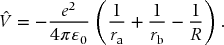Keeping track of these various constants can become cumbersome, which has motivated the use of atomic units (au) when performing quantum chemical calculations. Atomic units form a natural system of units when dimensions approach the atomic scale. These units are defined in Table 24.2. The atomic units for mass, charge and angular momentum are fairly obvious. The length scale is set by the Bohr radius a0, which is the diameter of the n = 1 state within the Bohr model of the atom. The energy scale is set by the Hartree Eh, which is the potential energy associated with the n = 1 state of the H atom. The unit of time t0 is equal to how long it would take an electron with the mean velocity of a 1s electron, which is the atomic unit of speed v0, to travel the length of one Bohr radius.

Rewriting the Hamiltonian in terms of atomic units of Hartree for the energy, the Bohr radius for length, and the charge and mass of the electron, we obtain

(24.28)The Schrödinger equation for H+2 can be solved analytically (exactly) by introducing confocal elliptical coordinates. Unfortunately, this solution cannot be extended to any other system with more than one electron because of electron correlation. Therefore, let us explore approximate answers to the H2+ problem that will be applicable and illustrative of what we can do for any diatomic molecule.

### 24.4.1 Directed practice

Write out the Hamiltonian using a form similar to Eq. (24.28), that is, using atomic units, for Li25+.

### 24.4.2 Molecular orbitals from linear combinations of atomic orbitals (LCAO-MO)

The idea here is that we are going to make an orbital for the system (a molecular orbital, MO), out of component orbitals that each atom brings to the system (the atomic orbitals). We will add (or subtract) the atomic orbitals and multiply them by factors (for normalization), but we will not raise them to powers. Therefore, the combinations of atomic orbitals are linear combinations. For the H+2 system we start off with the simplest approximation: The ground state is composed of a linear combination of a 1s orbital associated with the nucleus a, which we call A for short, and a 1s orbital from the nucleus b, which we call B for short.

There are two possible linear combinations (this should look pretty familiar from valence bond theory)

(24.29)(24.30)Normalization requires

(24.31)Each constituent orbital is normalized

(24.32)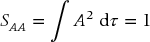(24.33)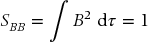But the overlap integral or overlap density will depend on the specific orbital combination and we call its value S

(24.34)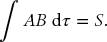The value of the overlap integral S depends on the internuclear separation R. Note that in Eqs. (24.31) and (24.34) we have made use of the identity that the overlap integral is symmetrical with respect to whether the integral is taken over the product AB or BA, that is, SAB = SBA = S. The normalization constant is then found to be

(24.35)Similarly

(24.36)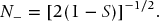#### 24.4.2.1 Directed practice

Evaluate the integrals in Eq. (24.31) to confirm the values of the normalization constant.

By looking at the squares of the wavefunctions, remembering that it is proportional to the probability distribution for the electron and noting that S is a positive number, we can interpret the meaning of the (+) and (–) combinations

(24.37)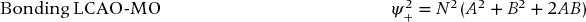(24.38)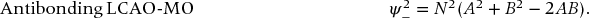The (+) combination enhances the electron density between the two nuclei and the (–) combination decreases the electron density in the internuclear region. Both combinations are cylindrically symmetric about the internuclear axis. As in valence bond theory, we identify this as σ symmetry. However, they are differentiated by their behavior under inversion. The (+) combination has gerade symmetry, denoted g, and the (–) combination has ungerade symmetry, denoted u. We will discover shortly that the bonding orbital is lower in energy than the antibonding orbital so the (+) combination is a σg MO and the (–) combination, which is the next lowest orbital of σ symmetry, is a σ*u MO, where the * denotes antibonding character. Since both orbitals are derived from a 1s configuration, we further label them as the 1σg and the 1σ*u. This naming convention is used for the molecular orbitals generated by homonuclear diatomics, for which the MOs are generated by degenerate orbitals of the same n value. Again, just as in valence bond theory, orbitals that are antisymmetric with respect to a plane of symmetry that includes the internuclear axis have π symmetry.

To develop our discussion beyond the phenomenological and the qualitative, we need to solve for the energies of these wavefunctions. We want to develop a method we can use more generally than for just the H2+ molecular ion. For this we use the variational theorem introduced in Chapter 20 and the matrix notation introduced in Chapter 22. First, we write the wavefunction as a linear combination of the 1s wavefunction centered on nucleus A and one centered on B as

(24.39)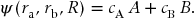The coefficients cA and cB will be determined in a variational calculation. The two 1s basis functions depend only on the electronic coordinates. They possess no dependence on the internuclear separation R. This R-dependence is to be found in the coefficients cA and cB.

With the Hamiltonian in Eq. (24.28) and the wavefunctions from Eq. (24.39), we calculate the expectation value of the total energy as a function of the internuclear distance R,

(24.40)The variational principle can be stated as

For a given Hamiltonian, the lowest energy is the energy given by the correct wavefunction. All other wavefunctions result in a higher energy.

Therefore, the values of cA and cB that minimize the energy at a given value of R from Eq. (24.40) represent the best possible wavefunction for the system in terms of the two basis functions that we have chosen.

We perform this minimization by solving two coupled equations for cA and cB. In solving this system of equations we will need the overlap integrals defined above, and the Hamiltonian integrals defined by

(24.41)The solution is obtained by finding the roots of the secular determinant

(24.42)